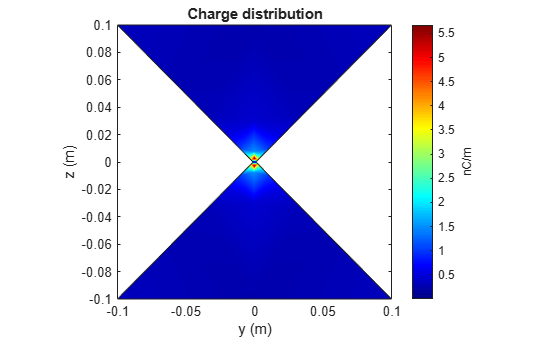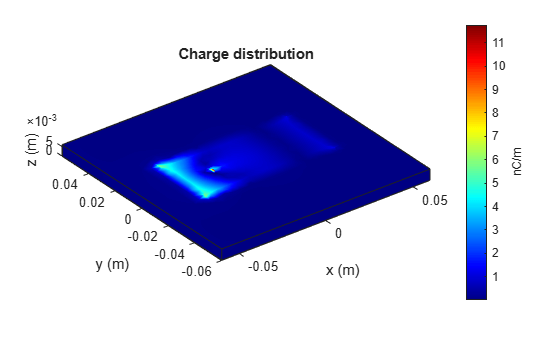# charge

Charge distribution on metal or dielectric antenna or array surface

## Syntax

``charge(object,frequency)``
``c = charge(object,frequency)``
``[c,p] = charge(object,frequency)``
``charge(object,frequency,'dielectric')``
``c = charge(object,frequency,'dielectric')``
``c = charge(___,Name,Value)``

## Description

example

````charge(object,frequency)` calculates and plots the absolute value of the charge on the surface of an antenna or array object surface at a specified frequency.```

example

````c = charge(object,frequency)` returns a vector of charges in C/m on the surface of an antenna or array object, at a specified frequency.```
````[c,p] = charge(object,frequency)` returns a vector of charges in C/m on the surface of an antenna or array object, at a specified frequency and at the point at which the charge calculation was performed.```

example

````charge(object,frequency,'dielectric')` calculates and plots the absolute value of charge at a specified frequency value on the dielectric face of the antenna or array.```
````c = charge(object,frequency,'dielectric')` returns the x, y, z components of the charge on the dielectric surface of an antenna or array object, at a specified frequency.```
````c = charge(___,Name,Value)` calculates the charge on the surface of an antenna using additional name-value pairs.```

## Examples

collapse all

Calculate and plot the charge distribution on a bowtieTriangular antenna at 70MHz frequency.

```h = bowtieTriangular; charge (h, 70e6);```Calculate charge distribution of linear array at 70 MHz frequency.

```h = linearArray; h.NumElements = 4; C = charge(h,70e6);```

Create a microstrip patch antenna using 'FR4' as the dielectric substrate.

```d = dielectric('FR4'); pm = patchMicrostrip('Length',75e-3, 'Width',37e-3, ... 'GroundPlaneLength',120e-3, 'GroundPlaneWidth',120e-3, ... 'Substrate',d)```
```pm = patchMicrostrip with properties: Length: 0.0750 Width: 0.0370 Height: 0.0060 Substrate: [1x1 dielectric] GroundPlaneLength: 0.1200 GroundPlaneWidth: 0.1200 PatchCenterOffset: [0 0] FeedOffset: [-0.0187 0] Tilt: 0 TiltAxis: [1 0 0] Load: [1x1 lumpedElement] ```
`show(pm)`Plot the charge distribution on the antenna at a frequency of 1.67 GHz.

```figure charge(pm,1.67e9,'dielectric')```Create a default pifa (planar inverted F antenna).

`ant = pifa;`

Visualize the charge distribution on the pifa antenna in log10 scale.

`charge(ant,1.75e9,'scale','log10')`## Input Arguments

collapse all

Antenna or array object, specified as a scalar handle.

Frequency used to calculate charge distribution, specified as a scalar in Hz.

Example: 70e6

Data Types: `double`

### Name-Value Pair Arguments

Specify optional comma-separated pairs of `Name,Value` arguments. `Name` is the argument name and `Value` is the corresponding value. `Name` must appear inside quotes. You can specify several name and value pair arguments in any order as `Name1,Value1,...,NameN,ValueN`.

Example: `'scale','log10'`

Scale to visualize the charge distribution on the surface of the antenna, specified as a string or a function handle. The string values are: `'linear'`, `'log'`, `'log10'`. By default, the value is `'linear'`. The function handle can be of any mathematical function such as `log`, `log10`, `cos`, or `sin`.

Data Types: `char` | `function_handle`

## Output Arguments

collapse all

Complex charges, returned as a 1-by-n vector in C/m. This value is calculated on every triangle mesh or every dielectric tetrahedron face on the surface of an antenna or array.

Cartesian coordinates representing the center of each triangle in the mesh, returned as a 3-by-n real matrix.